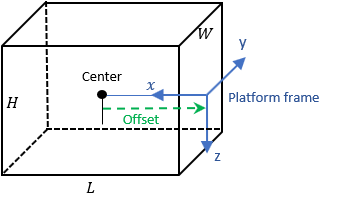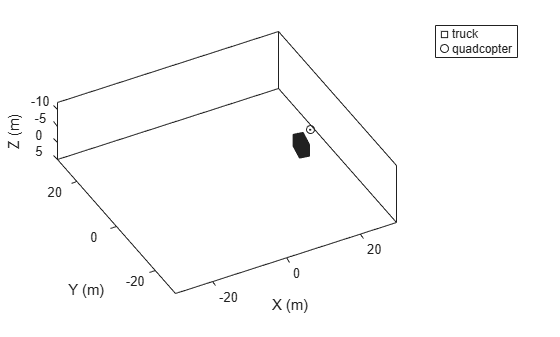# platform

## Syntax

```ptfm = platform(sc) ptfm = platform(sc,Name,Value) ```

## Description

`ptfm = platform(sc)` adds a `Platform` object, `ptfm`, to the tracking scenario, `sc`. The function creates a platform with default property values. Platforms are defined as points or cuboids with aspect-dependent properties. Each platform is automatically assigned a unique ID specified in the `platformID` field of the `Platform` object.

`ptfm = platform(sc,Name,Value)` adds a platform with additional properties specified by one or more `Name,Value` pair arguments. `Name` is a property name and `Value` is the corresponding value. `Name` must appear inside single quotes (`''`). You can specify several name-value pair arguments in any order as `Name1,Value1,...,NameN,ValueN`. Any unspecified properties take default values.

## Input Arguments

expand all

Tracking scenario, specified as a `trackingScenario` object.

### Name-Value Arguments

Specify optional pairs of arguments as `Name1=Value1,...,NameN=ValueN`, where `Name` is the argument name and `Value` is the corresponding value. Name-value arguments must appear after other arguments, but the order of the pairs does not matter.

Before R2021a, use commas to separate each name and value, and enclose `Name` in quotes.

Platform classification identifier specified as a nonnegative integer. You can define your own platform classification scheme and assign `ClassID` values to platforms according to the scheme. The value of `0` is reserved for an object of unknown or unassigned class.

Example: `5`

Data Types: `double`

Current position of the platform, specified as a 3-element vector of scalars.

• When the `IsEarthCentered` property of the scenario is set to `false`, the position is specified as a three element Cartesian state [`x`, `y`, `z`] in meters.

• When the `IsEarthCentered` property of the scenario is set to `true`, the position is specified as a three element geodetic state: `latitude` in degrees, `longitude` in degrees, and `altitude` in meters.

You should only specify position when creating a stationary platform. If you choose to specify the trajectory of the platform, do not use `Position`. Instead, use the `Trajectory` argument.

Data Types: `double`

Orientation of the platform, specified as a 3-element vector of scalars in degrees. The three scalars are the [`yaw`, `pitch`, `roll`] rotation angles from the local reference frame to the platform's body frame.

You should only specify `Orientation` when creating a stationary platform. If you choose to specify the orientation over time, use the `Trajectory` argument.

Data Types: `double`

Platform dimensions and origin offset, specified as a structure. The structure contains the `Length`, `Width`, `Height`, and `OriginOffset` of a cuboid that approximates the dimensions of the platform. The `OriginOffset` is the position vector from the center of the cuboid to the origin of the platform coordinate frame. The `OriginOffset` is expressed in the platform coordinate system. For example, if the platform origin is at the center of the cuboid rear face as shown in the following figure, then set `OriginOffset` as `[-L/2, 0, 0]`. The default value for `Dimensions` is a structure with all fields set to zero, which corresponds to a point model.Fields of `Dimensions`

FieldsDescriptionDefault
`Length`Dimension of a cuboid along the x direction`0`
`Width`Dimension of a cuboid along the y direction`0`
`Height`Dimension of a cuboid along the z direction`0`
`OriginOffset`Position of the platform coordinate frame origin with respect to the cuboid center`[0 0 0 ]`

Example: `struct('Length',5,'Width',2.5,'Height',3.5,'OriginOffset',[-2.5 0 0])`

Data Types: `struct`

Platform motion, specified as either a `kinematicTrajectory` object, a `waypointTrajectory` object, or a `geoTrajectory` object. The trajectory object defines the time evolution of the position and velocity of the platform frame origin, as well as the orientation of the platform frame relative to the scenario frame.

Platform signatures, specified as a cell array of `irSignature`, `rcsSignature`, and `tsSignature` objects or an empty cell array. The cell array contains at most only one instance for each type of signature objects listed. A signature represents the reflection or emission pattern of a platform such as its radar cross-section, target strength, or IR intensity.

A pose estimator, specified as a pose estimator object. The pose estimator determines platform pose with respect to the local NED scenario coordinate. The interface of any pose estimator must match the interface of `insSensor`. By default, pose estimator accuracy properties are set to zero.

Emitters mounted on the platform, specified as a cell array of emitter objects, such as `radarEmitter` or `sonarEmitter`.

Sensors mounted on platform, specified as a cell array of sensor objects such as `irSensor`, `fusionRadarSensor`, `monostaticLidarSensor`, or `sonarSensor`.

## Output Arguments

expand all

Scenario platform, returned as a `Platform` object.

## Examples

expand all

Create a tracking scenario and a platform following a circular path.

```scene = trackingScenario('UpdateRate',1/50); % Create a platform plat = platform(scene); % Follow a circular trajectory 1 km in radius completing in 400 hundred seconds. plat.Trajectory = waypointTrajectory('Waypoints', [0 1000 0; 1000 0 0; 0 -1000 0; -1000 0 0; 0 1000 0], ... 'TimeOfArrival', [0; 100; 200; 300; 400]); % Perform the simulation while scene.advance p = pose(plat); fprintf('Time = %f ', scene.SimulationTime); fprintf('Position = ['); fprintf('%f ', p.Position); fprintf('] Velocity = ['); fprintf('%f ', p.Velocity); fprintf(']\n'); end```
```Time = 0.000000 ```
```Position = [ ```
```0.000000 1000.000000 0.000000 ```
```] Velocity = [ ```
```15.707701 -0.000493 0.000000 ```
```] ```
```Time = 50.000000 ```
```Position = [ ```
```707.095476 707.100019 0.000000 ```
```] Velocity = [ ```
```11.107152 -11.107075 0.000000 ```
```] ```
```Time = 100.000000 ```
```Position = [ ```
```1000.000000 0.000000 0.000000 ```
```] Velocity = [ ```
```0.000476 -15.707961 0.000000 ```
```] ```
```Time = 150.000000 ```
```Position = [ ```
```707.115558 -707.115461 0.000000 ```
```] Velocity = [ ```
```-11.107346 -11.107341 0.000000 ```
```] ```
```Time = 200.000000 ```
```Position = [ ```
```0.000000 -1000.000000 0.000000 ```
```] Velocity = [ ```
```-15.707963 0.000460 0.000000 ```
```] ```
```Time = 250.000000 ```
```Position = [ ```
```-707.098004 -707.098102 0.000000 ```
```] Velocity = [ ```
```-11.107069 11.107074 0.000000 ```
```] ```
```Time = 300.000000 ```
```Position = [ ```
```-1000.000000 0.000000 0.000000 ```
```] Velocity = [ ```
```-0.000476 15.707966 0.000000 ```
```] ```
```Time = 350.000000 ```
```Position = [ ```
```-707.118086 707.113543 0.000000 ```
```] Velocity = [ ```
```11.107262 11.107340 0.000000 ```
```] ```
```Time = 400.000000 ```
```Position = [ ```
```-0.000000 1000.000000 0.000000 ```
```] Velocity = [ ```
```15.708226 -0.000493 0.000000 ```
```] ```

Create a tracking scenario with two cuboid platforms following circular trajectories.

```sc = trackingScenario; % Create the platform for a truck with dimension 5 x 2.5 x 3.5 (m). p1 = platform(sc); p1.Dimensions = struct('Length',5,'Width',2.5,'Height',3.5,'OriginOffset',[0 0 0]); % Specify the truck's trajectory as a circle with radius 20 meters. p1.Trajectory = waypointTrajectory('Waypoints', [20*cos(2*pi*(0:10)'/10)... 20*sin(2*pi*(0:10)'/10) -1.75*ones(11,1)], ... 'TimeOfArrival', linspace(0,50,11)'); % Create the platform for a small quadcopter with dimension .3 x .3 x .1 (m). p2 = platform(sc); p2.Dimensions = struct('Length',.3,'Width',.3,'Height',.1,'OriginOffset',[0 0 0]); % The quadcopter follows the truck at 10 meteres above with small angular delay. % Note that the negative z coordinates correspond to positive elevation. p2.Trajectory = waypointTrajectory('Waypoints', [20*cos(2*pi*((0:10)'-.6)/10)... 20*sin(2*pi*((0:10)'-.6)/10) -11.80*ones(11,1)], ... 'TimeOfArrival', linspace(0,50,11)');```

Visualize the results using `theaterPlot`.

```tp = theaterPlot('XLim',[-30 30],'YLim',[-30 30],'Zlim',[-12 5]); pp1 = platformPlotter(tp,'DisplayName','truck','Marker','s'); pp2 = platformPlotter(tp,'DisplayName','quadcopter','Marker','o'); % Specify a view direction and animate. view(-28,37); set(gca,'Zdir','reverse'); while advance(sc) poses = platformPoses(sc); plotPlatform(pp1, poses(1).Position, p1.Dimensions, poses(1).Orientation); plotPlatform(pp2, poses(2).Position, p2.Dimensions, poses(2).Orientation); end```## Version History

Introduced in R2018b